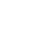# Create types with Semigroups

An introduction to concatting items via the formal Semi-group interface. Semi-groups are simply a type with a concat method that are associative. We define three semigroup instances and see them in action.Benji Shine
~ 3 years ago

Can you explain the `{x: y}` in the parameter to `concat`?

``````x,
concat: ({x: y}) =>
Sum(x + y),``````

Is that the same `x` as on the line above it?Benji Shine
~ 3 years ago

I think I understand, now that I've read the transcript:

I like to destructure this other Sum so why don't we say x and we'll assign it to y, and now we can just say x + y, much simpler. We are destructuring Sum type and just grabbing x off of it and calling it a y here. It should still work, and there we have it.

In the statement `lots = Sum(3).concat(50)` , the destructuring assignment makes the values be...

``````concat({x:  y}) => {
// x is 3 and y is 50
return Sum(3 + 50);
}),``````

This is just like saying:

`````` const { x: y } = { x: 50 };
// now y = 50``````